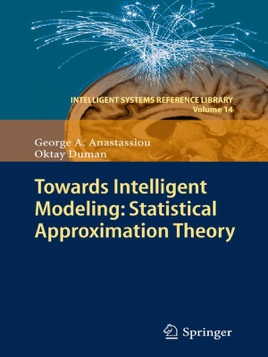• 134,99 €

## Descrizione dell’editore

The main idea of statistical convergence is to demand convergence only for a majority of elements of a sequence. This method of convergence has been investigated in many fundamental areas of mathematics such as: measure theory, approximation theory, fuzzy logic theory, summability theory, and so on. In this monograph we consider this concept in approximating a function by linear operators, especially when the classical limit fails. The results of this book not only cover the classical and statistical approximation theory, but also are applied in the fuzzy logic via the fuzzy-valued operators. The authors in particular treat the important Korovkin approximation theory of positive linear operators in statistical and fuzzy sense. They also present various statistical approximation theorems for some specific real and complex-valued linear operators that are not positive. This is the first monograph in Statistical Approximation Theory and Fuzziness. The chapters are self-contained and several advanced courses can be taught.

The research findings will be useful in various applications including applied and computational mathematics, stochastics, engineering, artificial intelligence, vision and machine learning. This monograph is directed to graduate students, researchers, practitioners and professors of all disciplines.